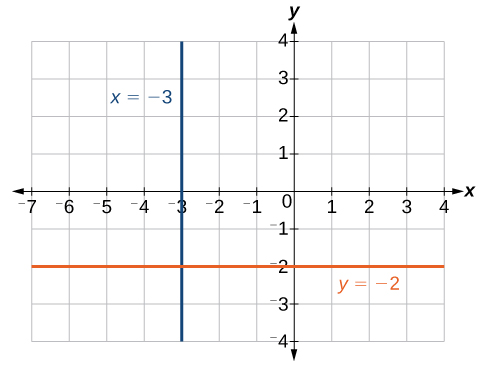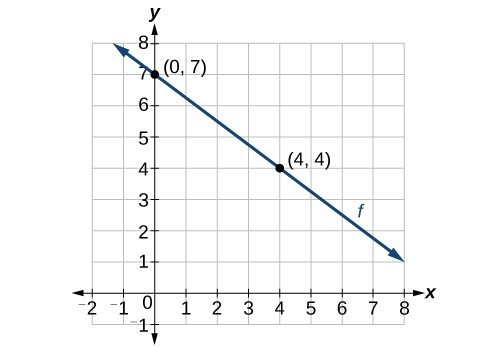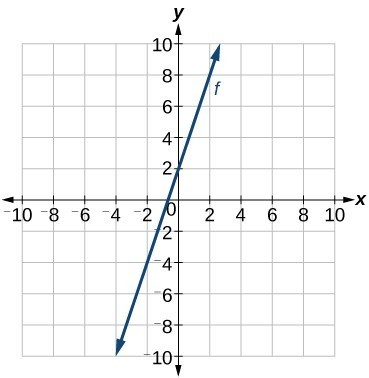## 4.2 – Equations of Linear Functions

### Learning Objectives

• (4.2.1) – Write the equation of a line using the point-slope formula
• Define the equation of a line given the slope and a point
• Define the equation of a line given two points
• (4.2.2) – Identify the equations for vertical and horizontal lines
• (4.2.3) – Given a graph, write the equation of a linear function
• Given two function values, write the equation of the linear function passing through them
• (4.2.4) – Model an Application With a Linear Function

In this section, we will learn how to write equations for linear functions given different pieces of information, including two points on the line and the graph of the line.  We will also identify the equations of horizontal and vertical lines and write linear equations from written information.

# (4.2.1) – Write the equation of a line using the point-slope formula

We have seen that we can define the slope of a line given two points on the line, and use that information along with the y-intercept to graph the line.  If you don’t know the y-intercept, or the equation for the line you can use two points to define the equation of the line using the point-slope formula:

$y-{y}_{1}=m\left(x-{x}_{1}\right)$

This is an important formula, as it will be used in other areas of college algebra and often in calculus to find the equation of a tangent line. We need only one point and the slope of the line to use the formula. After substituting the slope and the coordinates of one point into the formula, we simplify it and write it in slope-intercept form.

### The Point-Slope Formula

Given one point and the slope, the point-slope formula will lead to the equation of a line:

$y-{y}_{1}=m\left(x-{x}_{1}\right)$

### Define the equation of a line given the slope and a point

In our first example, we will start with the slope, then we will show how to find the equation of a line without being given the slope.

### Example

Write the equation of the line with slope $m=-3$ and passing through the point $\left(4,8\right)$. Write the final equation in slope-intercept form.

### Define the equation of a line given two points

In our next example we will start with two points and define the equation of the line that passes through them.

### Example

Find the equation of the line passing through the points $\left(3,4\right)$ and $\left(0,-3\right)$. Write the final equation in slope-intercept form.

The following video examples shows how to write the equation for a line given it’s slope and a point on the line.

# (4.2.2) – Identify the equations for vertical and horizontal lines

The equations of vertical and horizontal lines do not require any of the preceding formulas, although we can use the formulas to prove that the equations are correct. The equation of a vertical line is given as

$x=c$

where c is a constant. The slope of a vertical line is undefined, and regardless of the y-value of any point on the line, the x-coordinate of the point will be c.

Suppose that we want to find the equation of a line containing the following points: $\left(-3,-5\right),\left(-3,1\right),\left(-3,3\right)$, and $\left(-3,5\right)$. First, we will find the slope.

$\displaystyle m=\frac{5 - 3}{-3-\left(-3\right)}=\frac{2}{0}$

Zero in the denominator means that the slope is undefined and, therefore, we cannot use the point-slope formula. However, we can plot the points. Notice that all of the x-coordinates are the same and we find a vertical line through $x=-3$.

The equation of a horizontal line is given as

$y=c$

where c is a constant. The slope of a horizontal line is zero, and for any x-value of a point on the line, the y-coordinate will be c.

Suppose we want to find the equation of a line that contains the following set of points: $\left(-2,-2\right),\left(0,-2\right),\left(3,-2\right)$, and $\left(5,-2\right)$. We can use the point-slope formula. First, we find the slope using any two points on the line.

$\large \begin{array}{l}m=\frac{-2-\left(-2\right)}{0-\left(-2\right)}\hfill \\ =\frac{0}{2}\hfill \\ =0\hfill \end{array}$

Use any point for $\left({x}_{1},{y}_{1}\right)$ in the formula, or use the y-intercept.

$\begin{array}{l}y-\left(-2\right)=0\left(x - 3\right)\hfill \\ y+2=0\hfill \\ y=-2\hfill \end{array}$

The graph is a horizontal line through $y=-2$. Notice that all of the y-coordinates are the same.The line $x=−3$ is a vertical line. The line $y=−2$ is a horizontal line.

### Example

Find the equation of the line passing through the given points: $\left(1,-3\right)$ and $\left(1,4\right)$.

# (4.2.3) – Given a graph, write the equation of a linear function

Now that we have written equations for linear functions in both the slope-intercept form and the point-slope form, we can choose which method to use based on the information we are given. That information may be provided in the form of a graph, a point and a slope, two points, and so on. Look at the graph of the function f below.The function f passing through the points (0.7) and  (4,4) with a negative slope.

We are not given the slope of the line, but we can choose any two points on the line to find the slope. Let’s choose (0, 7) and (4, 4). We can use these points to calculate the slope.

$\large \begin{array}{l} m=\frac{{y}_{2}-{y}_{1}}{{x}_{2}-{x}_{1}}\hfill \\ \text{ }=\frac{4 - 7}{4 - 0}\hfill \\ \text{ }=-\frac{3}{4} \end{array}$

Now we can substitute the slope and the coordinates of one of the points into the point-slope form.

$\large \begin{array}{l} y-{y}_{1}=m\left(x-{x}_{1}\right)\hfill \\ y - 4=-\frac{3}{4}\left(x - 4\right)\hfill \end{array}$

If we want to rewrite the equation in the slope-intercept form, we would find

$\large \begin{array}{l} y - 4=-\frac{3}{4}\left(x - 4\right)\hfill \\ y - 4=-\frac{3}{4}x+3\hfill \\ \text{ }y=-\frac{3}{4}x+7\hfill \end{array}$Rewrite the equation in slope intercept form.

If we wanted to find the slope-intercept form without first writing the point-slope form, we could have recognized that the line crosses the y-axis when the output value is 7. Therefore, b = 7. We now have the initial value b and the slope m so we can substitute m and b into the slope-intercept form of a line.

So the function is $\displaystyle f\left(x\right)=-\frac{3}{4}x+7$, and the linear equation would be $\displaystyle y=-\frac{3}{4}x+7$.

### Given the graph of a linear function, write an equation to represent the function.

1. Identify two points on the line.
2. Use the two points to calculate the slope.
3. Determine where the line crosses the y-axis to identify the y-intercept by visual inspection.
4. Substitute the slope and y-intercept into the slope-intercept form of a line equation.

### Example

Write an equation for a linear function given a graph of f shown below.In the following video we show an example of how to write the equation of a line given it’s graph.

### Example

If f is a linear function, with $f\left(3\right)=-2$ , and $f\left(8\right)=1$, find an equation for the function in slope-intercept form.

In the video we show another example of how to write a linear function given two points written with function notation.

# (4.2.4) – Model an Application With a Linear Function

In the real world, problems are not always explicitly stated in terms of a function or represented with a graph. Fortunately, we can analyze the problem by first representing it as a linear function and then interpreting the components of the function. As long as we know, or can figure out, the initial value and the rate of change of a linear function, we can solve many different kinds of real-world problems.

### Given a linear function f and the initial value and rate of change, evaluate f(c).

1. Determine the initial value and the rate of change (slope).
2. Substitute the values into $f\left(x\right)=mx+b$.
3. Evaluate the function at $x=c$.

Initial value is a term that is typically used in applications of functions.  It can be represented as the starting point of the relationship we are describing with a function. In the case of linear functions, the initial value is typically the y-intercept. Here are some characteristics of initial value:

• The point $(0,y)$ is often the initial value of a linear function
• The y value of the initial value comes from b in the slope intercept form of a linear function, $f\left(x\right)=mx+b$
• The initial value can be found by solving for b, or substituting 0 for x in a linear function.

In our first example, we are given a scenario where Marcus wants to increase the number of songs in his music collection by a fixed amount each month. This is a perfect candidate for a linear function because the increase in the number of songs stays the same each month. We will identify the initial value for the music collection, and write an equation that represents the number of songs in the collection for any number of months, t.

### Example

Marcus currently has 200 songs in his music collection. Every month, he adds 15 new songs. Write a formula for the number of songs, N, in his collection as a function of time, t, the number of months. How many songs will he own in a year?

In our next example, we will show that you can write the equation for a linear function given two data points.  In this case, Ilya’s weekly income depends on the number of insurance policies he sells.  We are given his income for two different weeks and the number of policies sold.  We first find the rate of change and then solve for the initial value.

### Example

Working as an insurance salesperson, Ilya earns a base salary plus a commission on each new policy. Therefore, Ilya’s weekly income, I, depends on the number of new policies, n, he sells during the week. Last week he sold 3 new policies, and earned $760 for the week. The week before, he sold 5 new policies and earned$920. Find an equation for I(n), and interpret the meaning of the components of the equation.

In the following video example we show how to identify the initial value, slope and equation for a linear function.

We will show one more example of how to write a linear function that represents the monthly cost to run a company given monthly fixed costs and production costs per item.

### Example

Suppose Ben starts a company in which he incurs a fixed cost of $1,250 per month for the overhead, which includes his office rent. His production costs are$37.50 per item. Write a linear function where C(x) is the cost for x items produced in a given month.

## Summary

### Slope of a line

• The slope of a line indicates the direction in which a line slants as well as its steepness. Slope is defined algebraically as:$\displaystyle m=\frac{{y}_{2}-{y}_{1}}{{x}_{2}-{x}_{1}}$
• Given the slope and one point on a line, we can find the equation of the line using the point-slope formula. $y-{y}_{1}=m\left(x-{x}_{1}\right)$

### Equations of Lines

• Standard form of a line is given as $Ax+By=C$.
• The slope-intercept form of a line is given as $y=mx+b$
• The equation of a vertical line is given as $x=c$
• The equation of a horizontal line is given as $y=c$